# Simplifying Boolean Expression using K Map

### Minterm Solution of K Map

The following are the steps to obtain simplified minterm solution using K-map.
Step 1: Initiate
Express the given expression in its canonical form
Step 2: Populate the K-map
Enter the value of ‘one’ for each product-term into the K-map cell, while filling others with zeros.
Step 3: Form Groups

1. Consider the consecutive ‘ones’ in the K-map cells and group them (green boxes).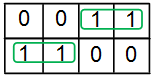2. Each group should contain the largest number of ‘ones’ and no blank cell.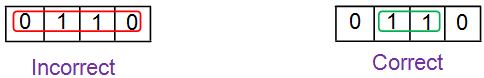3. The number of ‘ones’ in a group must be a power of 2 i.e. a group can contain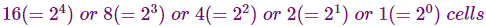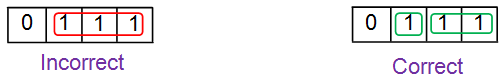4. Grouping has to be carried-on in decreasing order meaning, one has to try to group for 8 (octet) first, then for 4 (quad), followed by 2 and lastly for 1 (isolated ‘ones’).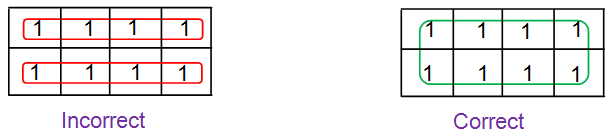5. Grouping is to done either horizontally or vertically or interms of squares or rectangles. Diagonal grouping of ‘ones’ is not permitted.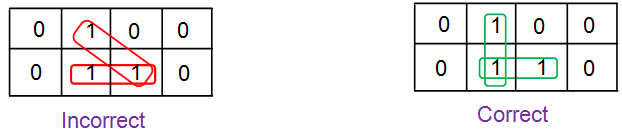6. The same element(s) may repeat in multiple groups only if this increases the size of the group.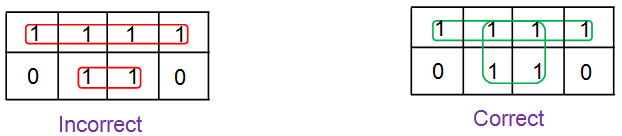7. The elements around the edges of the table are considered to be adjacent and can be grouped together.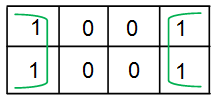8. Don’t care conditions are to be considered only if they aid in increasing the group-size (else neglected).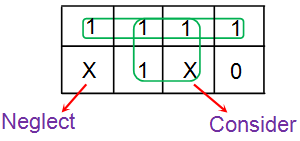Step 4: Obtain Boolean Expression for Each Group
Express each group interms of input variables by looking at the common variables seen in cell-labelling. For example in the figure shown below there are two groups with two and one number of ‘ones’ in them (Group 1 and Group 2, respectively). All the ‘ones’ in the Group 1 of the K-map are present in the row for which A = 0. Thus they contain the variable A̅. Further these two ‘ones’ are present in adjacent columns which have only B term in common as indicated by the pink arrow in the figure.

Hence the next term is B. This yields the product term corresponding to this group as A̅B. Similarly the ‘one’ in the Group 2 of the K-map is present in the row for which A = 1. Further the variables corresponding to its column are B̅C̅. Thus one gets the overall product-term for this group as AB̅C̅.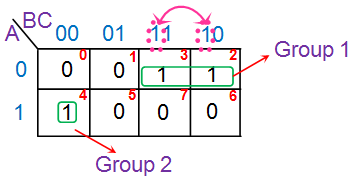Step 5: Obtain Boolean Expression for the Output
The product-terms obtained for individual groups are to be combined to form sum-of-product (SOP) form which yields the overall simplified Boolean expression. This means that for the K-map shown in Step 4, the overall simplified output expression is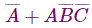A few more examples elaborating K-map simplification process are shown below.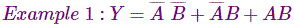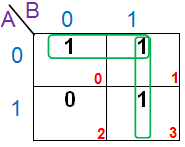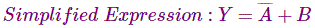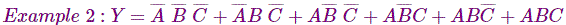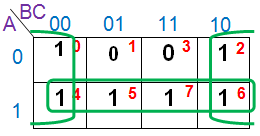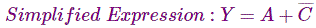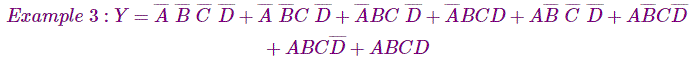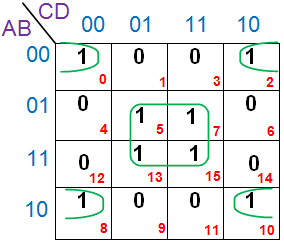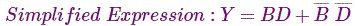### Maxterm Solution of K Map

The method to be followed in order to obtain simplified maxterm solution using K-map is similar to that for minterm solution except minor changes listed below.

1. K-map cells are to be populated by ‘zeros’ for each sum-term of the expression instead of ‘ones’.
2. Grouping is to be carried-on for ‘zeros’ and not for ‘ones’.
3. Boolean expressions for each group are to be expressed as sum-terms and not as product-terms.
4. Sum-terms of all individual groups are to be combined to obtain the overall simplified Boolean expression in product-of-sums (POS) form.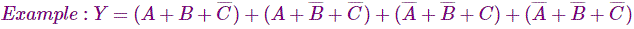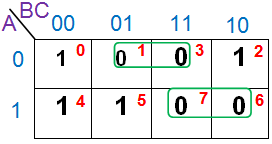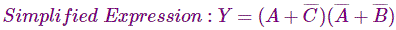Want To Learn Faster? 🎓
Get electrical articles delivered to your inbox every week.
No credit card required—it’s 100% free.